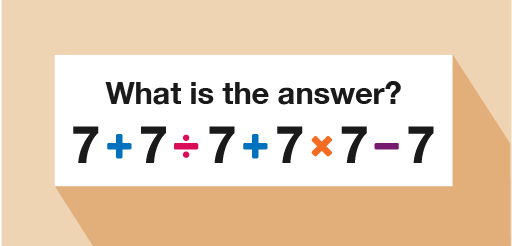Everyday maths 2 (Wales)

Start this free course now. Just create an account and sign in. Enrol and complete the course for a free statement of participation or digital badge if available.

Free course

# 11 FormulasFigure 27 Formulas

Before diving in to this topic, you first need to learn about the order in which you need to carry out addition, subtraction, multiplication and division. Have you ever seen a question like the one below posted on social media?Figure 28 A calculation using the four operations

There are usually a wide variety of answers given by various people. But how is it possible that such a simple calculation could cause so much confusion? It’s all to do with the order in which you carry out the calculations.

• If you go from left to right:
• 7 + 7 = 14
• 14 ÷ 7 = 2
• 2 + 7 = 9
• 9 × 7 = 63
• 63 − 7 = 56

Check this on a calculator and you will see that the correct answer is actually 50. How do you arrive at this answer? You have to use the correct order of operations, sometimes called BIDMAS.

FSM_2_CYMRU